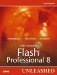# The Transform Class

### The transform Class

The TRansform class allows you to collect information about color transformations and matrixes applied to movie clips.

It is important that when using this class to import it first at the beginning of your script like this:

` import flash.geom.Transform; `

To instantiate a new instance of the transform class, use this code as a template:

` var myTrans:Transform = new Transform(mc); `

• mc The movie clip that the transform is being applied to.

#### Properties

##### colorTransform

Availability: FP:8, AS:1.0

Generic Template: mytrans.colorTransform;

Description:

This property is a ColorTransform object used to adjust colors of movie clips.

Example:

This example will create a new instance of the transform object and send it to the Output panel:

`[View full width] import flash.geom.Transform; var trans:Transform = new Transform(this); trace(trans.colorTransform);  //output: (redMultiplier=1, greenMultiplier=1, blueMultiplier=1, alphaMultiplier=1,redOffset=0, greenOffset=0, blueOffset=0, alphaOffset=0) `

##### concatenatedColorTransform

Availability: FP:8, AS:1.0

Generic Template: mytrans.concatenatedColorTransform;

Description:

This property is the combined ColorTransform objects used to adjust colors of movie clips.

Example:

This example will create a new instance of the transform object, set its color transform, and output it:

`[View full width] import flash.geom.Transform; import flash.geom.ColorTransform; var trans:Transform = new Transform(this); trace(trans.colorTransform);  var myColorTrans:ColorTransform = new ColorTransform(1, 3, 6, 1, 0, 0, 255, 0); trans.colorTransform = myColorTrans; trace(trans.concatenatedColorTransform); //output: (redMultiplier=1, greenMultiplier=1, blueMultiplier=1, alphaMultiplier=1,redOffset=0, greenOffset=0, blueOffset=0, alphaOffset=0) //(redMultiplier=1, greenMultiplier=3, blueMultiplier=6, alphaMultiplier=1, redOffset=0,greenOffset=0, blueOffset=255, alphaOffset=0) `

##### concatenatedMatrix

Availability: FP:8, AS:1.0

Generic Template: mytrans.concatenatedMatrix;

Description:

This property is the combined Matrix objects used to adjust positions of movie clips.

Example:

This example will create a new instance of the transform object, set its matrix, and output it:

`[View full width] import flash.geom.Transform; import flash.geom.Matrix; //create the matrix var myMatrix:Matrix = new Matrix(); myMatrix.rotate(45); var trans:Transform = new Transform(this); trace(trans.matrix);  trans.matrix = myMatrix; trace(trans.concatenatedMatrix); //output: (a=1, b=0, c=0, d=1, tx=0, ty=0) //(a=0.525321960449219, b=0.850903511047363, c=-0.850903511047363, d=0.525321960449219,tx=0, ty=0) `

##### matrix

Availability: FP:8, AS:1.0

Generic Template: myTRans.matrix;

Description:

This property is the Matrix object used to adjust positions of movie clips.

Example:

This example will create a new instance of the TRansform object and output its matrix property:

` import flash.geom.Transform; var trans:Transform = new Transform(this); trace(trans.matrix);  //output: (a=1, b=0, c=0, d=1, tx=0, ty=0) `

##### pixelBounds

Availability: FP:8, AS:1.0

Generic Template: myTRans.pixelBounds;

Description:

Defines the bounding rectangle of the movie clip being used.

Example:

This example will create a new instance of the transform object and output its pixelBounds property:

` import flash.geom.Transform; var trans:Transform = new Transform(this); trace(trans.pixelBounds); //output: (x=33554431, y=33554431, w=0, h=0) `Macromedia Flash Professional 8 Unleashed
ISBN: 0672327619
EAN: 2147483647
Year: 2005
Pages: 319